# GSEB Solutions Class 9 Maths Chapter 9 Areas of Parallelograms and Triangles Ex 9.4

Gujarat Board GSEB Solutions Class 9 Maths Chapter 9 Areas of Parallelograms and Triangles Ex 9.4 Textbook Questions and Answers.

## Gujarat Board Textbook Solutions Class 9 Maths Chapter 9 Areas of Parallelograms and Triangles Ex 9.4

Question 1.
Parallelogram ABCD and rectangle ABEF are on the same base AB and have equal areas. Show that the perimeter of the parallelogram is greater than that of the rectangle.
Solution:
Given: Parallelogram ABCD and rectangle ABEF are on the same base AB and have equal areas.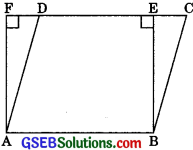To prove: The perimeter of the parallelogram ABCD is greater than that of rectangle ABEF. Proof: Here the parallelogram ABCD and the rectangle ABEF are on the same base AB and between the same parallels AB and FC. Then, perimeter of the parallelogram ABCD = 2(AB + AD)
and, the perimeter of the rectangle ABEF = 2(AB + AF).

∴ ∠ADF is an acute angle. (< 90°) [Angle sum property of a triangle ] ⇒ ∠AFD > ∠ADF
The side opposite to the greater angle of a triangle is longer.
⇒ AB + AD > AB + AF
⇒ 2(AB + AD) > 2(AB + AF)
∴ Perimeter of the parallelogram ABCD > Perimeter of the rectangle ABEF.Question 2.
In figure, D and E are two points on BC such that BD = DE = EC. Show that ar(ΔABD) = ar(ΔADE) = ar(ΔAEC). Can you now answer the question that you have left in the ‘Introduction, of this chapter, whether the field of Budhia has been actually divided into three parts of equal area?[Remark: Note that by taking BD = DE = EC, the triangle ABC is divided into three triangles ABD, ADE and AEC of equal areas. In the same way, by dividing BC into n equal parts and joining the points of division so obtained to the opposite vertex of BC, you can divide ΔABC into n triangles of equal areas.]
Solution:
Let AM ⊥ BC. Then, the height of each of ΔABD, ΔADE and ΔAEC is the same.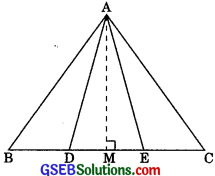∴ ar(ΔABD) = $$\frac {1}{2}$$ x BD x AM ……(1)
ar(ΔADE) = $$\frac {1}{2}$$ x DE x AM ……(2)
and, ar(ΔAEC) = $$\frac {1}{2}$$ x EC x AM ……(3)
∴ BD = DE = EC
∴ From (1), (2) and (3),
Yes, the field of Budhia has been actually divided into three parts of equal area.

Question 3.
In figure, ABCD, DCFE and ABFE are parallelograms. Show that
Solution:
∴ ABCD is a || gm.
[Opposite sides of a || gm are equal Again, DCFE is a || gm.]
∴ DE || CF ……(2)
[Opposite sides of a || gm are equal Also, ABFE is a || gm]
∴ AE = BF ……(3)
[Opposite sides of a || gm are equal From (1), (2) and (3)]
ΔADE ≅ ΔBCF  [By SSS congruency]
∴ Two congruent triangles are equal in areas.Question 4.
In the figure, ABCD is a parallelogram, and BC is produced to a point Q such that AD = CQ. If AQ intersect DC at P, show that ar(ΔBPC) = ar(ΔDPQ).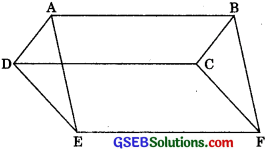Solution:
Join AC.
∴ AQAC and AQDC are on the same base QC and between the same parallels AD and QC.
∴ ar(ΔQAC) = ar(ΔQDC) ……(1)
Two triangles on the same base (or equal bases) and between the same parallels are equal in areas.
⇒ ar(ΔQAC) ≅ ar(ΔQPC) = ar(ΔQDC) ≅ ar(ΔQPC)
Subtracting the same areas from both sides.
⇒ ar(ΔPAC) = ar(ΔQDP) ……(2)
∴ ΔPAC and ΔPBC are on the same base PC and between the same parallels AB and DC.
∴ ar(ΔPAC) = ar(ΔPBC) …….(3)
Two triangles on the same base (or equal bases) and between the same parallels are equal in area.
From (2) and (3), we get
ar(ΔPBC) = ar(ΔQDP)
∴ ar(ΔBPC) = ar(ΔDPQ)Question 5.
In figure, ABC and BDE are two equilateral triangles such that D is the mid-point of BC. If AE intersect BC at F, show that:
(i) ar(ΔBDE) = $$\frac {1}{4}$$ ar(ΔABC)
(ii) ar(ΔBDE) = $$\frac {1}{2}$$ ar(ΔBAE)
(iii) ar(ΔABC) = 2ar(ΔBEC)
(iv) ar(ΔBFE) = ar(ΔAFD)
(v) ar(ΔBFE) = 2ar(ΔFED)
(vi) ar(ΔFED) = $$\frac {1}{8}$$ ar(AAFC)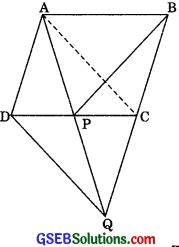[Hint: Join EC and AD. Show that BE || AC and DE || AB, etc.]
Solution:
∴ ΔABC is an equilateral triangle.
∴ ∠ABC = ∠BCA = ∠CAB = 60° ……(1)
∴ ΔBDE is an equilateral triangle.
∴ ∠BDE = ∠DEB = ∠EBD = 60° ……(2)
⇒ ∠ABC = ∠BDE
AB || DE …….(3)
∴ Alternate interior angles. Similarly, ∠ACB = ∠EBC
∴ AC || BE …….(4) (each 60°)
Now, ΔCBA and ΔCEA are on the same base AC and between the same parallels.
∴ ar(ΔCBA) = ar(ΔCEA)
Two triangles on the same base (or equal bases) and between the same parallels are equal in area.
⇒ ar(ΔABC) = ar(ΔCDA) + ar(ΔCED) + ar(ΔADE) …….(5)
In ΔABC, AD is a medan.
∴ ar(ΔABD) = ar(ΔACD) = $$\frac {1}{2}$$ ar(ΔABC) …….(6)
A median of a triangle divides it into two triangles of equal area.

In ΔEBC, ED is a median.
∴ ar(ΔECD) = ar(ΔEBD) = $$\frac {1}{2}$$ ar(ΔEBC) ……(7)
∴ A median of a triangle divides it into two triangles of equal area.
∴ ΔDEA and ADBE are on the same base DE and between the same parallels AB and DE.
∴ ar(ΔDEA) = ar(ΔDBE) ………(8)
∴ Two triangles on the same base (or equal bases) and between the same parallels are equal in area.
Using (6), (7) and (8), in (5) gives
ar(ΔABC) = $$\frac {1}{2}$$ ar(ΔABC) + ar(ΔBDE) + ar(ΔBDE)
⇒ $$\frac {1}{2}$$ ar(ΔABC) = 2ar(ΔBDE)
⇒ ar(ΔBDE) = $$\frac {1}{4}$$ ar(ΔABC)(ii) ∴ ΔBAE and ΔBCE are on the same base BE and between the same parallels BE and AC.
∴ ar(ΔBAE) = ar(ΔBCE)
[∴ Two triangles on the same base (or equal bases) and between the same parallels are equal in area]
⇒ $$\frac {1}{2}$$ ar(ΔBAE) = 2ar(ΔBDE) | From (7)
⇒ ar(ΔBDE) = $$\frac {1}{4}$$ ar(ΔBAE)

(iii) 2ar(ΔBEC) = 2ar(ΔBDE) [From (7)]
= 4ar(ΔBDE)
= ar(ΔABC) [From (i)]

(iv) ∴ ΔEBD and ΔEAD are on the same base ED and between the same parallels AB and DE.
[∴ Two triangles on the same base (or equal bases) and between the same parallels are equal in area]
⇒ ar(ΔEBD) – ar(ΔEFD) = ar(ΔEAD) – ar(ΔEFD)
[Subtracting the same areas from both sides]
⇒ ar(ΔBFE) = ar(ΔAFD)

(v) ar(ABDE) = $$\frac {1}{4}$$ ar(ΔABC)  [From (i)]
= $$\frac {1}{4}$$ .2 ar(ΔABD)
= $$\frac {1}{4}$$ ar(ΔABD)
∴ Bases of ΔBDE and ΔABD are the same.
∴ Altitude of ABDE = $$\frac {1}{2}$$ Altitude of ΔABD ……..(9)
ar(ΔBEF) = ar(ΔAFD) ……..(10) [From (iv)]
But Altitude of ΔBDE = Altitude of ΔBEF (Considering common vertex)
And, altitude of ΔABD = Altitude of ΔAFD (Considering common vertex A)
Altitude of ΔBEF = $$\frac {1}{2}$$ Altitude of ΔAFD ………(11)
From (10) and (11),
BF = 2FD ……(12)
In ΔBFE and ΔFED,
∴ BF = 2FD
and alt. (ΔBFE) = alt. (ΔFED)
∴ ar(ΔBFE) = 2ar(ΔFED).

(vi) Let the altitude of ΔABD be h.
Then, altitude of ΔBED = $$\frac {h}{2}$$ [From (9)]
Now, ar(ΔFED) = $$\frac {1}{2}$$. FD. $$\frac {h}{2}$$ = $$\frac {FD.h}{4}$$ …….(13)
ar(ΔAFC) = $$\frac {1}{2}$$ . FC .h
= $$\frac {1}{2}$$ (FD + DC) h 2
= $$\frac {1}{2}$$ (FD + BD) h 2
= $$\frac {1}{2}$$ (FD + BF + FD) h 2
= $$\frac {1}{2}$$ (2FD + BF) h 2
= $$\frac {1}{2}$$ (2FD + 2FD) h [From (12) ]
= 2 . FD . h ……(14)
From (13) and (14), we obtain
ar(ΔFED) = $$\frac {1}{8}$$ ar(ΔAFC)Question 6.
Diagonals AC and BD of a quadrilateral ABCD intersect each other at P. Show that
ar(ΔAPB) x ar(ΔCPD) = ar(ΔAPD) x ar(ΔBPC)
[Hint: From A and C, draw perpendiculars to BD]
Solution:
Construction: From A and C, draw perpendiculars AE and CF respectively to BD.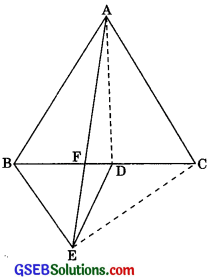Proof: ar(ΔAPB) x ar(ΔCPD)
= $$\frac {(PB) (AE)}{2}$$ x $$\frac {(DP x CE)}{2}$$= $$\frac {1}{4}$$ (PB) (AE) (DP) (CF) ……..(1)
ar(ΔAPD) x ar(ΔBPC)
= $$\frac {(DP) (AE)}{2}$$  = $$\frac {(PB) (CF)}{2}$$
= $$\frac {1}{4}$$ (PB) (AE) (DP) (CF) ……(2)
From (1) and (2), we get
ar(ΔAPB) x ar(ΔCPD)
= ar(ΔAPD) x ar(ΔBPC).Question 7.
P and Q are respectively the mid-points of sides AB and BC of a triangle ABC and R is the mid-point of AP. Show that:
1. ar(APRQ) = $$\frac {1}{2}$$ ar(ΔARC)
2. ar(ARQC) = $$\frac {3}{8}$$ ar(ΔABC)
3. ar(ΔPBQ) = ar(ΔARC)
Solution:
Construction: Join AQ and CP.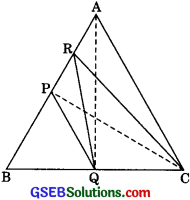Proof: 1.  ar(ΔPRQ) = ar(ΔARQ)
(∴ a median of a A divides it into two triangles of equal area)
= $$\frac {1}{2}$$ ar(ΔAPQ) = $$\frac {1}{2}$$ ar(ΔBPQ)
= $$\frac {1}{2}$$ ar(ΔCPQ) = $$\frac {1}{2}$$ . $$\frac {1}{2}$$ ar(ΔBPC)
= $$\frac {1}{4}$$ ar(ΔBPC) = $$\frac {1}{4}$$ . $$\frac {1}{2}$$ ar(ΔABC)
= $$\frac {1}{8}$$ ar(ΔABC) ………(1)
$$\frac {1}{2}$$ ar(ΔARC) = $$\frac {1}{2}$$ . $$\frac {1}{2}$$ ar(ΔAPC)
= $$\frac {1}{4}$$ ar(ΔAPC) = $$\frac {1}{4}$$.$$\frac {1}{2}$$ ar(ΔABC)
= $$\frac {1}{8}$$ar(ΔABC) ………..(2)

From (1) and (2), we have
ar(ΔPRQ) = $$\frac {1}{2}$$ ar(ΔARC)2. ar(ΔRQC) = ar(ΔRBQ)
(∴ a median of a A divides it into triangles of equal areas)
= ar(ΔPRQ) + ar(ΔBPQ)
= $$\frac {1}{8}$$ ar(ΔABC) + $$\frac {1}{2}$$ ar(ΔPBC) [Using (1)]
= $$\frac {1}{8}$$ ar(ΔABC) + $$\frac {1}{2}$$.$$\frac {1}{2}$$ ar(AABC)
= $$\frac {1}{8}$$ ar(ΔABC) + $$\frac {1}{4}$$ ar(ΔABC)
= $$\frac {3}{8}$$ ar(ΔABC)

3. ar(ΔPBQ) = $$\frac {1}{4}$$ ar(ΔABC) …….(3) [From (ii)]
ar(ΔARC) = $$\frac {1}{4}$$ ar(ΔABC) …….(4) [From (i) ]
From (3) and (4), we get ar(ΔPBQ) = ar(ΔARC)

Question 8.
In figure, ABC is a right triangle right angled at A. BCDE, ACFG and ABMN are squares on the sides BC, CA and AB respectively. Line segment AX ⊥ DE meets BC at Y. Show that: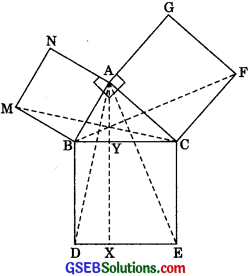(i) ΔMBC – ΔABD
(ii) ar(BYXD) = 2ar(ΔMBC)
(iii) ar(BYXD) = ar(ABMN)
(iv) ΔFCB = ΔACE
(v) ar(CYXE) = 2ar(ΔFCB)
(vi) ar(CYXE) = ar(ACFG)
(vii) ar(BCED) = ar(ABMN) + ar(ACFG).
Note: Result (vii) is the famous theorem of Pythagoras. You shall learn a simpler proof of this theorem in class X.
Solution:
(i) In ΔMBC and ΔABD,
MB = AB …(1) [Sides of a square]
BC = BD …(2) [Sides of a square]
∠MBA = ∠CBD [Each = 90°]
⇒ ∠MBA + ∠ABC = ∠CBD + ∠ABC
⇒ ∠MBC = ∠ABD …….(3)
In view of (1), (2) and (3),
⇒ ΔMBC = ΔABD [By congruence SAS rule]

(ii) ar(BYXD) = 2 ar(ΔABD) (∴ BD || AX and common base is BD)
= ar(BYXD) = 2ar(ΔMBC)
[From part (i), ar(ΔMBC) = ar(ΔABD)](iii) ∴ ar(BYXD) = 2ar(ΔABD)
and ar(ΔBMN) = 2ar(ΔMBC)
(∴ MB || NC, and common base is MB)
= 2ar(ΔABD) | From (i)
∴ ar(BYXD) = ar(ABMN)

(iv) In ΔFCB and ΔACE,
FC = AC [Sides of a square]
CB = CE [Sides of a square]
∠FCA = ∠BCE [Each = 90°]
⇒ ∠FCA + ∠ACB = ∠BCE + ∠ACB
(Adding the same on both sides)
⇒ ∠FCB = ∠ACE
∴ ΔFCB = ΔACE [SAS congruence rule]

(v) ar(CYXE) = 2ar(ΔACE) = 2ar(ΔFCB) [From (iv)]
∴ AFCB = AACE
∴ ar(AFCB) = ar(AACE)
Congruent As have equal areas

(vi) ∴ ar(CYXE) = 2ar(AACE) = 2ar(AFCB) And, ar(ACFG) = 2ar(AFCB)
(∴ BG || CF and CF is the common base)
∴ ar(CYXE) = ar(ACFG)

(vii) ar(BCED = ar(CYXE) + ar(BYXD)
= ar(ACFG) + ar(ABMN) [From (iii) and (vi)]
= ar(ABMN) + ar(ACFG)